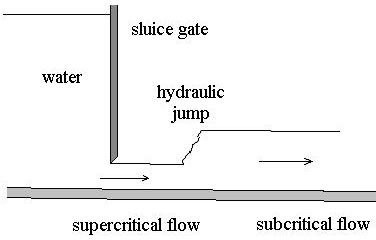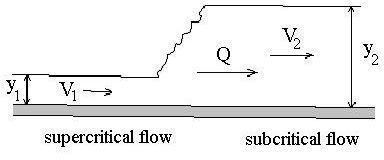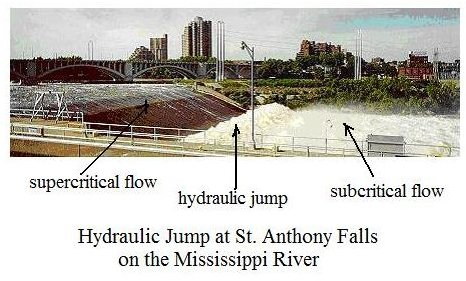# The Hydraulic Jump as a Transition from Subcritical to Supercritical Flow in Open Channel Flow

Page content

## Review of Supercritical flow and Subcritical Flow

In order to discuss the hydraulic jump, it’s necessary to refer to supercritical flow and subcritical flow, so these two types of open channel flow will be reviewed briefly. Supercritical flow is characterized by high flow velocity, shallow depth of flow, and relatively steep channel bottom slope. Subcritical flow, on the other hand, is characterized by low flow velocity, high depth of flow, and relatively small channel bottom slope. Supercritical flow occurs for Froude Number (Fr) greater than 1, and subcritical flow occurs for Froude number less than 1, where Fr = V/(gy)1/2, and V is the average velocity in the channel, y is the depth of flow, and g is the acceleration due to gravity.

## Where Does a Hydraulic Jump Occur

A hydraulic jump occurs wherever a supercritical flow is taking place in a channel thatdoesn’t have a slope steep enough to maintain the supercritical flow. The hydraulic jump provides a transition to subcritical from supercritical flow.Two physical situations that would give rise to a hydraulic jump are shown in the diagrams. The one on the left is a transition from a steep channel slope with supercritical flow to a smaller slope that will only sustain a subcritical flow. The diagram on the right shows a supercritical flow coming under a sluice gate on a slope that will only sustain a subcritical flow. In both cases a hydraulic jump is present to provide an abrupt transition from supercritical to subcritical flow. Open channel flow can transition smoothly from subcritical to supercritical flow, but there is no way for a smooth transition from supercritical to subcritical flow to take place in open channel flow.

## Equation Across a Hydraulic JumpThrough the use of the three fluid mechanics conservation equations (the energy equation, the continuity equation, and the momentum equation), the following equation can be derived relating the conditions before a hydraulic jump to the condition after the jump. The diagram at the left shows the parameters in the equation.y2/y1 = (1/2)[-1 + (1 + 8Fr12)1/2] (eqn 1)

where Fr1 = V1/(y1g)1/2, and

y1 = depth of flow before the hydraulic jump, ft

y2 = depth of flow after the hydraulic jump, ft

V1 = average flow velocity before the hydraulic jump, ft/sec

V2 = average flow velocity after the hydraulic jump, ft/sec

## Head Loss Across a Hydraulic Jump

Due to the turbulence in the hydraulic jump, there will be frictional head loss across the jump. The amount of head loss may sometimes be of interest and is given by the following equation:

hL = y1 + V12/2g - (y2 + V22/2g)

Rearranging this equation and substituting the expression for Fr1, leads to the following equation for hL in terms of the two depths of flow and the upstream Froude Number:

hL/y1 = 1 - y2/y1 + (Fr12/2)[1 - (y1/y2)2] (eqn 2)

## Example Calculations

Consider a hydraulic jump in a 2 ft wide rectangular channel. The flow rate through the channel is 20 cfs and the depth of flow before the jump is 1 ft. What will be the depth of flow after the hydraulic jump and what will be the head loss across the jump?

Solution: First calculate the flow velocity in the channel before the jump from V = Q/A = 20/(2x1) = 10 ft/sec. Second calculate the upstream Froude number, Fr1. Then you are ready to calculate y2 from eqn 1 and head loss, hL, from eqn 2.

Fr1 = 10/(1x32.2)1/2 =1.76

From eqn 1: y2 = (1)(1/2)[-1 +(1 + 8*1.762)1/2] = 2.03 ft

From eqn 2: hL = 1 - 2.03/1 + (1.762/2)[1 - (1/2.03)2] = 0.143 ft

For an Excel spreadsheet template for making hydraulic jump calculations, see the article, “Use of Excel Formulas for Hydraulic Jump Calculations.”

## References and Image Credits

1. Munson, B. R., Young, D. F., & Okiishi, T. H., Fundamentals of Fluid Mechanics, 4th Ed., New York: John Wiley and Sons, Inc, 2002.

2. Chow, V. T., Open Channel Hydraulics, New York: McGraw-Hill, 1959.

3. Bengtson, Harlan H. Open Channel Flow II - Hydraulic Jumps and Supercritical and Nonuniform Flow - An online, continuing education course for PDH credit.

Image credits:

1. Hydraulic Jump drawings - Drawn by H. Bengtson

2. Hydraulic Jump picture - Wikipedia Commons, StAnthonyFalls apron

## This post is part of the series: Open Channel Flow Basics

Open channel flow takes place with a free surface open to the atmosphere, so flow is due to gravity, rather than due to pressure as in pipe flow. The Manning equation applies for uniform flow. A hydraulic jump is nonuniform flow. It occurs for supercritical flow on a subcritical channel slope.